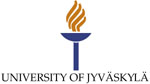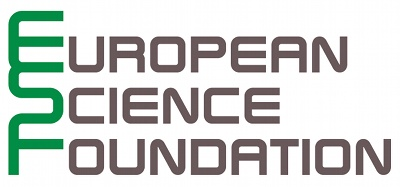The 5th Summer School on Mathematical Analysis, Advanced Methods for Partial Differential Equations and Optimization for junior scientists is organized by the Mathematical Information Technology Department of the University of Jyvaskyla (Finland) and the Euler International Mathematical Institute (Saint-Petersburg).

This year summer school is divided into two parts. It is possible to participate both events, or only a single event.

# Aims and scopes

The goal of this meeting is to get together young researchers and internationally known scientists working on mathematical, numerical and optimization methods for mathematical models based on on differential equations.

Main topics:

• mathematical analysis of models based on partial differential equations: solution theories, asymptotic analysis, and a priori estimates
• new mathematical models in applied science and engineering
• advanced numerical methods for partial differential equations: approximation theory, rate convergence estimates, a posteriori error estimates, error indicators, and adaptivity
• optimization methods for models based on partial differential equations.

This workshop belongs to the sequence of events:

The 1st SCOMA Workshop on Advanced Computer Simulation Methods for PhD students and junior scientists

The 2nd Workshop on Advanced Computer Simulation Methods for PhD students and junior scientists

The 3rd Workshop on Advanced Methods for Partial Differential Equation Analysis for junior scientists, 2010, Koivumiemi

The 4th Workshop on Advanced Methods for Partial Differential Equation Analysis for junior scientists, 2010, St.Petersburg